Function Repository Resource:

# GeodesicGridPoints

Generate points on a geodesic grid

Contributed by: Jan Mangaldan
 ResourceFunction["GeodesicGridPoints"][n] attempts to arrange n points in a geodesic grid on a unit sphere centered at the origin.

## Details and Options

A geodesic grid is also known as the icosahedral-hexagonal grid.
A geodesic grid subdivides a sphere according to the vertices of a geodesic polyhedron.
ResourceFunction["GeodesicGridPoints"] returns 10m2+2 points, where m=Ceiling[Sqrt[(n-2)/10]].
The points returned by ResourceFunction["GeodesicGridPoints"] are arranged to have the symmetries of an icosahedron.
ResourceFunction["GeodesicGridPoints"][n,WorkingPrecisionp] gives a result with entries of precision p.

## Examples

### Basic Examples (1)

Generate 162 geodesic grid points:

 In:=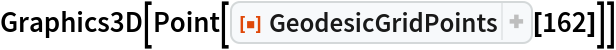Out=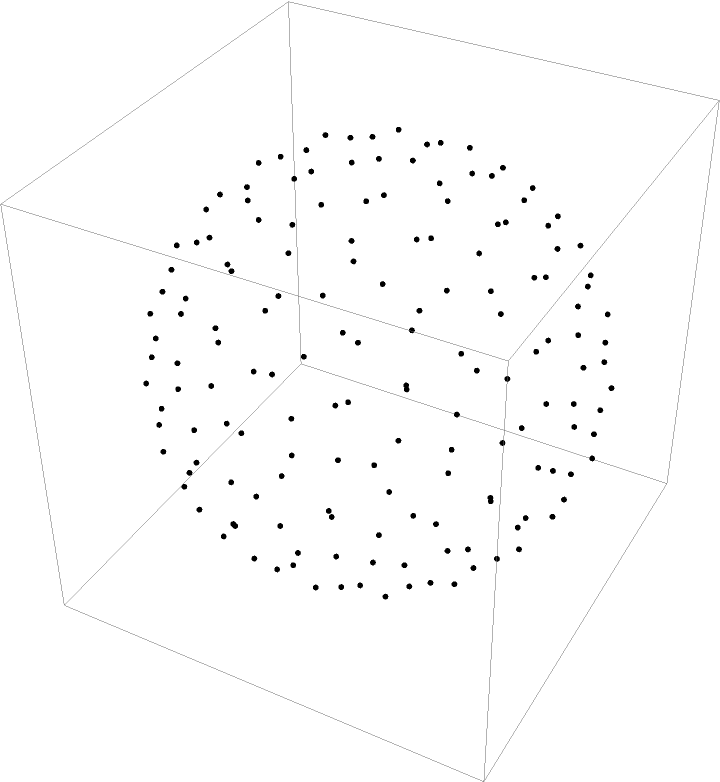### Options (2)

#### WorkingPrecision (2)

Generate machine-precision geodesic grid points:

 In:=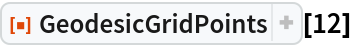Out=Generate geodesic grid points with 24-digit precision:

 In:=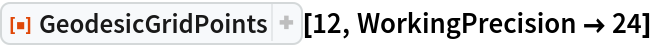Out=### Applications (1)

Use a Delaunay mesh to make an approximation to a sphere:

 In:=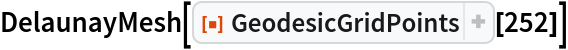Out=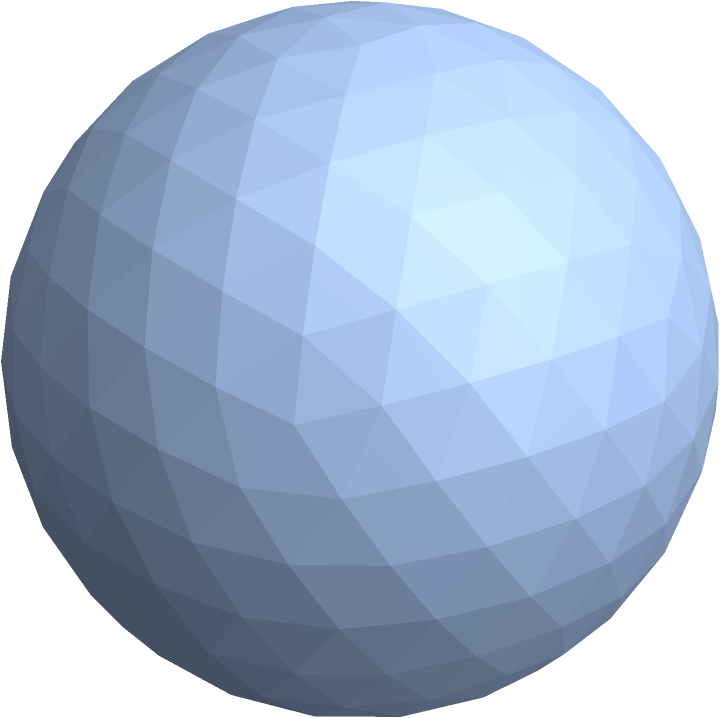### Neat Examples (1)

Visualize the spherical Voronoi diagram of a set of geodesic grid points:

 In:=Out=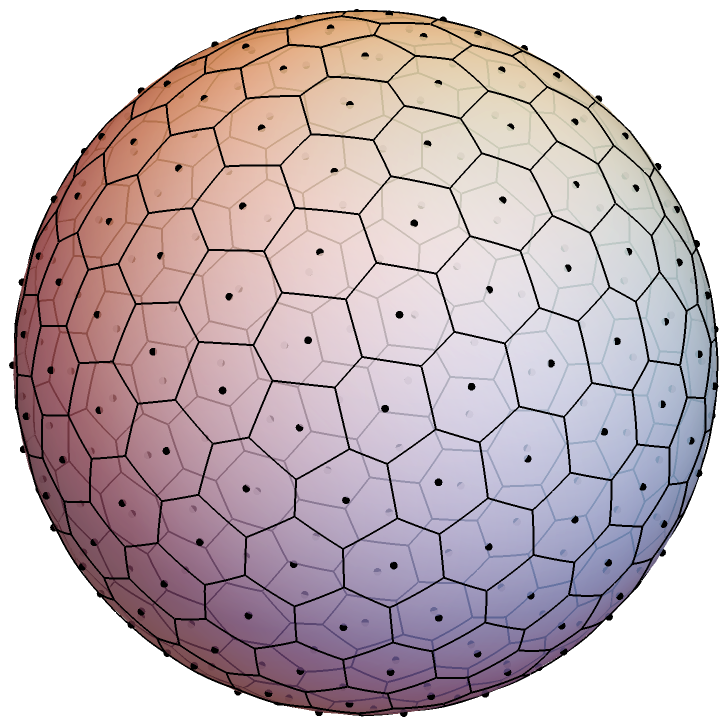## Version History

• 1.0.0 – 14 June 2021